Courses

# Revisal Problems (Past 13 Year) JEE Advanced (P Block Elements)

## 30 Questions MCQ Test Chemistry for JEE Advanced | Revisal Problems (Past 13 Year) JEE Advanced (P Block Elements)

Description
This mock test of Revisal Problems (Past 13 Year) JEE Advanced (P Block Elements) for JEE helps you for every JEE entrance exam. This contains 30 Multiple Choice Questions for JEE Revisal Problems (Past 13 Year) JEE Advanced (P Block Elements) (mcq) to study with solutions a complete question bank. The solved questions answers in this Revisal Problems (Past 13 Year) JEE Advanced (P Block Elements) quiz give you a good mix of easy questions and tough questions. JEE students definitely take this Revisal Problems (Past 13 Year) JEE Advanced (P Block Elements) exercise for a better result in the exam. You can find other Revisal Problems (Past 13 Year) JEE Advanced (P Block Elements) extra questions, long questions & short questions for JEE on EduRev as well by searching above.
QUESTION: 1

### Direction (Q. Nos. 1-10) This section contains 10 multiple choice questions. Each question has four choices (a), (b), (c) and (d), out of which ONLY ONE is correct. Q.  The number of unpaired electrons present in the species [Fe(H2O)5NO]2+ which is formed during 'brown ring’ test is

Solution:

In this, iron has +1 and NO has +1 oxidation state. Iron has 3 unpaired electrons with magnetic moment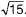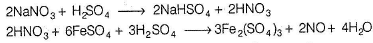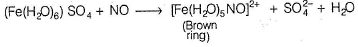QUESTION: 2

### In which one of the following pairs the species have similar geometry?

Solution:

Both have tetrahedral geometry.

QUESTION: 3

### O2 is converted into O+2 using

Solution: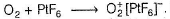PtF6 is one of the few oxidising agents sufficiently powerful to oxidise O2

QUESTION: 4

Which of the following pairs has the highest difference in their first ionisation energy?

Solution:

The pair of Ne and Na has the highest difference in their first ionisation energy.

QUESTION: 5

Among the compounds I to IV, those which hydrolyse easily
I. NCI3
II. NF3
III. BiCl3
IV. PCI3

Solution:

NF3 does not react with water at ambient temperature however, slowly hydrolysed by basic solution at 100°C

QUESTION: 6

Among the following pairs, those in which both species have similar structure are

Solution:

Both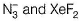are linear.

QUESTION: 7

The oxoacid of sulphur that does not contain S—S bond is

Solution: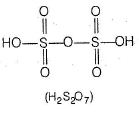QUESTION: 8

Among the following NO, SO2, SO3, CaO, Cl2O , Cl2O7, CO, N2O3, P4O10 the number of anhydrides of acids are

Solution:

SO2, SO3, Cl2O , Cl2O7, N2O3, P4O10 are acid anhydrides.

QUESTION: 9

How many H+ ions are required when the equation below is balanced with the smallest whole number coefficients?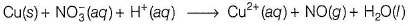Solution: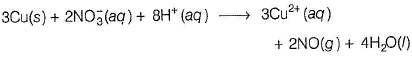QUESTION: 10

In the reaction,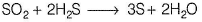Solution:

Here, oxidation state of hydrogen (+1) remains constant and oxygen (- 2) remains constant. But S in SO2 reduces from S (+ 4) to S(0) and sulphur in H2S oxidises from S(-2) to S (0).

*Multiple options can be correct
QUESTION: 11

Direction (Q. Nos. 11-20) This section contains 10 multiple choice questions. Each question has four choices (a), (b), (c) and (d), out of which ONE or MORE THAN ONE are correct.

Q.

Among the following, the species having same bond order are

Solution:

NO and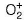have 15 electrons each. A ccordin g to MOT, the bond order in them is 2.5.

*Multiple options can be correct
QUESTION: 12

The oxidation number of sulphur atoms in Na2S4O6 are

Solution:

Two of the sulphur atoms present in the tetrathionate anion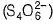a re in oxidation state 0 and two are in oxidation state +5

*Multiple options can be correct
QUESTION: 13

Which of the following statement is correct?

Solution:

(a) ONCl has 8+7+17 = 32 electrons;

ONO- has 8+7+8+1 = 24 electrons; Hence they are not isoelectronic.

(b) Molecular structure of ozone is not linear but appear bent.(c) Ozone is mild blue in gas state, dark blue in liquid and violet black in solid state.

(d) Ozone has all electrons paired. Hence a diamagnetic gas.

Ozone is sp2-hybridised, has bent structure and is diamagnetic in nature.

*Multiple options can be correct
QUESTION: 14

Among PbS, CuS, MnS, NiS, CoS, HgS, Ag2S, Bi2S3 and SnS2 the black coloured sulphides are

Solution:

CuS and Ag2S are black while CdS is yellow and MnS is flesh coloured.

*Multiple options can be correct
QUESTION: 15

Choose the correct statement from the following.

Solution:

In HF, there is strong intermolecular H-bonding between F and H. Hence, HF is lower volatile hydride.

*Multiple options can be correct
QUESTION: 16

The number of lone pairs are identical in the pairs.

Solution: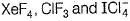have two lone pairs each.

*Multiple options can be correct
QUESTION: 17

Which of the following is oxidised byozone ?

Solution:

Ozone does not oxidise KMnO4 as Mn is already in maximum +7 oxidation state.

*Multiple options can be correct
QUESTION: 18

Identify the correct statements.

Solution:

Explanation : a) N-O bond in N-O2 is shorter than in NO3 because bond length increases with decreasing bond order and the N-O bond order in NO3 is less than the N-O bond order in NO2 .

b) NH3 or PH3 accepts a proton, an additional N−H or P−H bond is formed.

H3N: + H+ → NH + 4

H3N: + H+ → PH + 4

Due to the bigger size of P than N,P−H bond thus formed is much weaker than the N−H bond. Thus, NH3 has higher proton affinity than PH3.

c) S atom in SF4 is surrounded by only 4 Fluorine atoms whereas in SF6 the S atom is surrounded by 6 Fluorine atom. Due to highly stabilized of sp3 d2 configuration in SF6 the water molecules find less gap to attack on central atom. ... That's why SF4 is easily hydrolysed but SF6 is not hydrolysed.

d) Under ordinary conditions sulphur exists as S8 in solid state. In vapour state sulphur partly exists as S2 molecule and S2 molecule has two unpaired electrons in the antibonding π* orbitals. Hence, in vapour state sulphur exhibits paramagnetism.

*Multiple options can be correct
QUESTION: 19

Which of the following statements is/are correct ?

Solution:

(a) The Hlformed initially is oxidised by H2SO4 into iodine.
(b) Both have pyramidal geometry.
(c) Due to inert pair effect, Bi5+ gains two electrons and is converted into Bi3+.
(d) NH3 is not linear while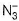is linear.

*Multiple options can be correct
QUESTION: 20

Regarding S8 molecule correct statements are

Solution:

S8 molecule has non-planar cyclic structure.

QUESTION: 21

Direction (Q. Nos. 21 and 22) This section contains a paragraph, each describing theory, experiments, data, etc. Two questions related to the paragraph have been given. Each question has only one correct answer among the four given options (a), (b), (c) and (d).

Passage

The reactions of Cl2 gas with cold dilute and hot cone. NaOH in water give sodium salts of two (different) oxoacids of chlorine, P and Q respectively. The Cl2 gas reacts with SO2 gas in the presence of charcoal, to give product R. R reacts with white phosphorus to give a compounds. On hydrolysis, S gives an oxoacid of phosphorus, T.

Q.

P and Q respectively are the sodium salts of

Solution: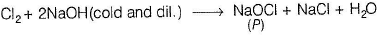NaOCI is sodium hypochlorite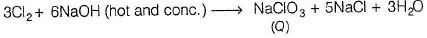NaCIO3 is sodium chlorate.

QUESTION: 22

The reactions of Cl2 gas with cold dilute and hot cone. NaOH in water give sodium salts of two (different) oxoacids of chlorine, P and Q respectively. The Cl2 gas reacts with SO2 gas in the presence of charcoal, to give product R. R reacts with white phosphorus to give a compounds. On hydrolysis, S gives an oxoacid of phosphorus, T.

Q.

R, S and T respectively are

Solution: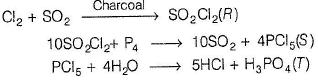QUESTION: 23

Direction (Q. No. 23) This section is based on Statement I and Statement II. Select the correct answer from the codes given below.

Q.

Statement I : The most stable octaatomic interhalogen compound is IF7.

Statement II : The difference between electronegativities of iodine and fluorine is greatest among the halogens.

Solution:
QUESTION: 24

Match the following reactions given in Column I with the corresponding products given in Column II.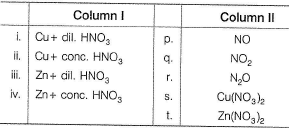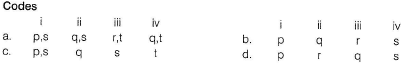Solution: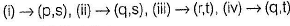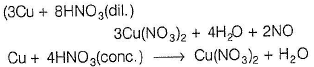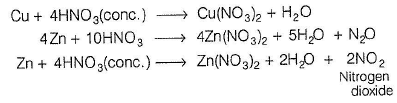*Answer can only contain numeric values
QUESTION: 25

Direction (Q. Nos. 25-30) This section contains 6 questions. When worked out will result in an integer from 0 fo 9 (both inclusive).

Q. How many moles of water reacts with one mole of PCI5 for complete hydrolysis?

Solution: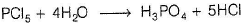*Answer can only contain numeric values
QUESTION: 26

The num ber of P—O—P bon ds in the cyclic trimer of metaphosphoric acid is

Solution: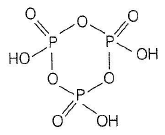*Answer can only contain numeric values
QUESTION: 27

Find the number of chemical species having pyramidal shape among these species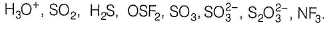Solution: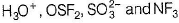have pyramidal shape.

*Answer can only contain numeric values
QUESTION: 28

Among the following the number of metals which are rendered passive in the presence of cone. HNO3,

Al, Sn, Pb, Fe, Cr, Zn, Ni, Hg, Cu.

Solution:

Al, Fe, Cr, Ni are rendered passive by cone. HNO3.

*Answer can only contain numeric values
QUESTION: 29

Find the number of chem ical species among following that have dπ - pπ bonds in their structure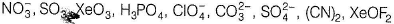Solution: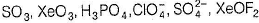have dπ - pπ bonding.

*Answer can only contain numeric values
QUESTION: 30

How many orbitals are involved in the hybridisation of iodine in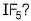Solution:

sp3d2-hybridisation.